Orbits round the Earth

An object projected horizontally near the Earth’s surface follows a parabolic trajectory. As the velocity of projection increases, there will be an occasion in which the trajectory follows the curvature of the Earth’s surface. If air resistance is negligible, the object will orbit round the Earth and will never meet the Earth’s surface.speed of cannonball at 0 km/hr launched horizontally from newton's very tall mountain, resulting in trajectory that hits Earth surface.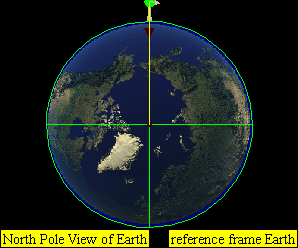speed of cannonball at 1 000 km/hr launched horizontally from newton's very tall mountain, resulting in trajectory that hits Earth surface.speed of cannonball at 2 000 km/hr launched horizontally from newton's very tall mountain, resulting in trajectory that hits Earth surface.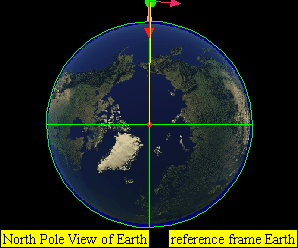speed of cannonball at 3 000 km/hr launched horizontally from newton's very tall mountain, resulting in trajectory that hits Earth surface.speed of cannonball at 4 000 km/hr launched horizontally from newton's very tall mountain, resulting in trajectory that hits Earth surface.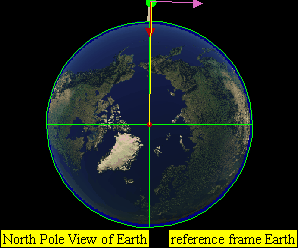speed of cannonball at 5 000 km/hr launched horizontally from newton's very tall mountain, resulting in trajectory that hits Earth surface.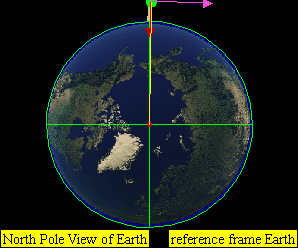speed of cannonball at 6 000 km/hr launched horizontally from newton's very tall mountain, resulting in trajectory that hits Earth surface.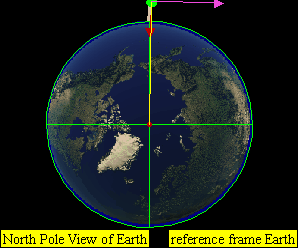speed of cannonball at 7 000 km/hr launched horizontally from newton's very tall mountain, resulting in trajectory that does NOT hit the Earth surface.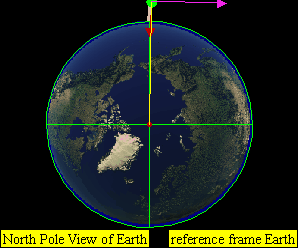speed of cannonball at 7 300 km/hr launched horizontally from newton's very tall mountain, resulting in trajectory orbit that does NOT hit the Earth surface that is a approximately a circular orbit.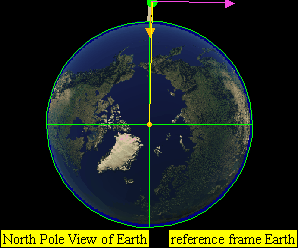speed of cannonball at 8 000 km/hr launched horizontally from newton's very tall mountain, resulting in trajectory orbit that does NOT hit the Earth surface.speed of cannonball at 9 000 km/hr launched horizontally from newton's very tall mountain, resulting in trajectory orbit that does NOT hit the Earth surface.speed of cannonball at 11 200 km/hr launched horizontally from newton's very tall mountain, resulting in trajectory that does NOT hit the Earth surface and escape from the Earth's gravitational pullplot of kinetic energy$E_{K}$, gravitational potential energy,$E_{P}$ and total Energy$E$versus distance away from centre of earth, r at R= Re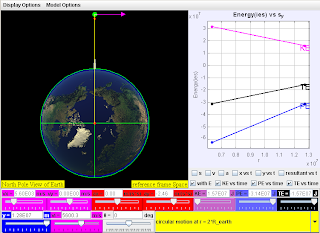plot of kinetic energy$E_{K}$, gravitational potential energy,$E_{P}$ and total Energy$E$versus distance away from centre of earth, r at R= Re, R= 2*Re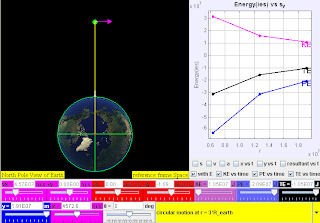plot of kinetic energy$E_{K}$, gravitational potential energy,$E_{P}$ and total Energy$E$versus distance away from centre of earth, r at R= Re, R= 2*Re, R=3*Replot of kinetic energy$E_{K}$, gravitational potential energy,$E_{P}$ and total Energy$E$versus distance away from centre of earth, r at R= Re, R= 2*Re, R=3*Re and lastly R = geostationary radius